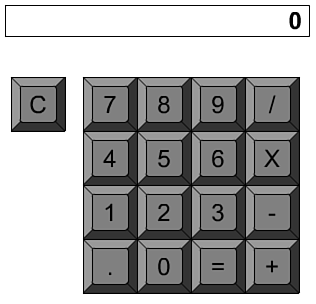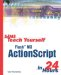I l @ ve RuBoard

The example movie 11calculator.fla re-creates a basic calculator like one you might buy at the store for a few dollars or the one that comes as an accessory program on your computer.

Now, let's re-create this movie by adding the scripts one at a time.

1. Start with the example movie 11calculator-noscripts.fla. This looks like Figure 11.2. There are the 10 digit keys, one key for each operation, an = key, and a decimal point. There is also a C key for clearing the calculator. The characters are superimposed on top of the keys, which are all instances of the same movie clip. A large dynamic text field at the top is linked to the variable display .

##### Figure 11.2. This simple calculator comes alive with ActionScript.2. Inside each movie clip is a single button. This button is the only other element in the library. The button should have a script attached to it. To do this, edit the Key movie clip and select the button. Now, add this script:

` on (release) {     _parent.keyPressed(this._name); } `

Whenever the button is pressed, the function keyPressed is called one level up, which is the root level. It is sent the name of the movie clip, so if the button is in an instance of a movie clip named Lucy, Lucy gets passed into keyPressed .

3. Back at the root level, each movie clip should have a unique name. Note that all the number keys are named after the digit they represent, so the 5 key is named 5. The same is true for the "." key. The rest of the movie clips are named plus, minus, multiply, divide, equals, and clear.

4. The rest of the scripts belong in the main movie frame. The first function clears out the display variable and sets many other variables . This function is also called immediately when the movie first runs. You will see that as the first line of the script:

` // start by clearing the display and variables clearAll(); function clearAll() {     display = "0"; // the visible display     memory = 0; // the buffer     operation = "none"; // what operation is happening     newNum = true; // whether to start a new number } `

The display variable is a string linked to what the user sees. It starts off with a "0" in it because typical calculators display that when they are turned on.

The memory variable holds the previous contents of the display window. If you think about how a calculator works, you will see why this is necessary. If you type 7, then +, and then 5, 7 disappears to be replaced by the 5. The 7 is held in memory as the user completes the next portion of the operation.

The operation also needs to be held in memory. If the user presses 7, then +, and then 5, the operation does not yet take effect. Then the user presses = or perhaps + again. At this point, the calculator must recall the first + and perform the operation between the 7 in memory and the 5 in the display.

The newNum flag is used to determine when the user's next keystroke starts a new number. For instance, if you type 7, then +, then 5, and then =, the answer, 12, displays. The next number you type, such as a 3, starts a new calculation.

5. The next portion of the program is the function that each button calls when it is pressed.

This function uses a switch structure in place of a series of if, then, else statements. It works the same way, but the variable is presented in the switch command, and the alternate values for the variable are presented in subsequent case statements. Each case segment must end in a break command. A catchall default statement is last and executes if none of the values match.

` // function called by buttons function keyPressed(keyName) {     // do something different for different keys     switch (keyName) {     case "clear" : // for clear key, start over         clearAll();         break;     case "plus" : // for any operation, go to operate function         operate(keyName);         break;     case "minus" :         operate(keyName);         break;     case "multiply" :         operate(keyName);         break;     case "divide" :         operate(keyName);         break;     case "equals" :         operate(keyName);         break;     default : // all others are added as part of the number         if (newNum) { // new numbers replace the display             display = keyName;             newNum = false;             if (display == "0") newNum = true; // don't allow leading 0s         } else {             display += keyName; // append a digit         }         break;     } } `

If the user presses the C key, the clearAll function is called to reset the calculator. If the user presses any operation key, the function operate is called. We'll get to that function next.

If the user presses any other key, such as a digit or the "." key, the digit either replaces the display variable or is appended to the end of it.

6. The operate function performs the actual calculations. It looks at the operation previously requested and performs that between the previous number and the current one. it uses parseFloat to convert the string in display to a number.

The none operation happens when the first number is entered after a clear. In this case, the memory " is simply replaced by the display . The four normal operations use += structures to perform the operations; memory now holds the result.

The operation variable remembers the new operation for next time. If it is equals , the none operation is stored.

Finally, the memory variable, which holds the result, is displayed. The newNum flag is set to true so that if the user starts typing a new number, it replaces the current display instead of appending itself to the end of it.

` // perform operation and prepare for next operation function operate(keyName) {     switch (operation) {     case "none" : // this is the first number         memory = parseFloat(display); // set memory to that number         break;     case "plus" : // perform operation with memory and new number         memory += parseFloat(display);         break;     case "minus" :         memory -= parseFloat(display);         break;    case "multiply" :         memory *= parseFloat(display);         break;     case "divide" :         memory /= parseFloat(display);         break;     }     // equals operation is like a clear, but results are displayed     if (keyName == "equals") {         operation = "none";     }  else {         operation = keyName; // remember this operation for next time     }     display = memory.toString(); // display result     newNum = true; // prepare for next number } `

This calculator program has some tricky parts to it. If you are having trouble, try playing computer. Walk through the code in a typical sequence of key presses such as 1+2-7= to see how memory , display , and operate change.

 I l @ ve RuBoardSams Teach Yourself Flash MX ActionScript in 24 Hours
ISBN: 0672323850
EAN: 2147483647
Year: 2002
Pages: 272